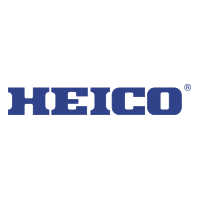# HEICO Corp NYSE:HEIHEICO Corp
NYSE:HEI
Price: 175.9 USD +2.85%
Updated:

## DCF Value

This DCF valuation model was created byAlpha Spread and was last updated on Dec 3, 2023.

Estimated DCF Value of oneHEI stock is 68.6 USD. Compared to the current market price of 175.9 USD, the stock is Overvalued by 61%.

DCF Valuation FAQ:
HEI DCF Value
Base Case
68.6 USD
Overvaluation 61%
DCF Value
Price68.6
DCF Value
HEICO Corp Competitors:
DCF Valuation
NPK
National Presto Industries Inc
NOC
Northrop Grumman Corp
LHX
L3harris Technologies Inc

Revenue
3.6B 4.8B
Operating Income
744m 1B
FCFF
674m 842m

## Present Value Calculation

The first step in the DCF calculation process is to estimate the company's future free cash flow. Once free cash flow is forecasted, it is discounted at a risk-appropriate discount rate. The resulting value is the present value of the company's free cash flow. You can change any model inputs below.

Available Valuation Models
1 valuation model availableUpdated on Dec 3, 2023
Default Valuation
Other investors haven't shared any valuation models for HEICO Corp.
Model Settings
Operating Model
Discount Rate
8.05%
Forecast Period
5 Years
Terminal Growth
0%
Discount Rate
8.05%
Terminal Growth
0%
Growth Period
5 Years

### DCF Model Base Case ScenarioDCF Model
Currency: USD
Millions

To view the process of deriving the DCF Value of one share from the estimated Present Value, see the DCF Value Calculation block.

## DCF Value Calculation

Depending on which type of operating model is chosen (equity or whole firm valuation model), the resulting value is either the value of equity or the value of the entire firm. In the case of the latter, to move from the value of the firm to the value of equity, liabilities are subtracted and assets are added.

### Capital Structure From Present Value to DCF ValuePresent Value 10.2B USD + Cash & Equivalents 694m USD Firm Value 10.9B USD - Debt 1.2B USD - Minority Interest 389m USD Equity Value 9.3B USD / Shares Outstanding 135m HEI DCF Value 68.6 USD
Overvalued by 61%

To view the process of calculating the Present Value of HEICO Corp' future free cash flow, see the Present Value Calculation block.

Discover More

## What is the DCF value of one HEI stock?

Estimated DCF Value of oneHEI stock is 68.6 USD. Compared to the current market price of 175.9 USD, the stock is Overvalued by 61%.

The true DCF Value lies somewhere between the worst-case and best-case scenario values. This is because the future is not predetermined, and the stock's DCF Value is based almost entirely on the future of the company. Knowing the full range of possible stock DCF values gives a complete picture of the investment risks and opportunities.

## How was the DCF Value calculated?

1. Present Value Calculation. Using the DCF Operating Model we projectHEICO Corp's future free cash flow and discount it at a selected discount rate to calculate its Present Value (10.2B USD).

2. DCF Value Calculation. We use the company's capital structure to calculate the total Equity Value based on the previously computed Present Value of the free cash flow. Dividing the Equity Value by the number of shares outstanding gives us the DCF Value of 68.6 USD per oneHEI share.